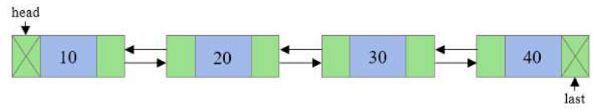# Golang program to count the number of nodes in a doubly linked list.

## Examples## Approach

Step 1 − Define a method that accepts the head of a doubly linked list.

Step 2 − Initialize temp:=head, count:=0

Step 3 − Iterate temp until it becomes nil.

Step 4 − Increase count by 1.

Step 5 − At the end, print count.

## Example

Live Demo

package main
import "fmt"
type Node struct {
prev *Node
value int
next *Node
}
func CreateNewNode(value int) *Node{
var node Node
node.next = nil
node.value = value
node.prev = nil
return &node
}
// Forward Traversal
count := 0
for temp!= nil{
fmt.Printf("%d ", temp.value)
temp = temp.next
count++
}
fmt.Printf("\nTotal number of nodes: %d", count)
}
func main(){
// 10 <−> 20 <−> 30 <−> 40
node_2 := CreateNewNode(20)
node_3 := CreateNewNode(30)
node_4 := CreateNewNode(40)
}
Doubly Linked List: 10 20 30 40
Total number of nodes: 4